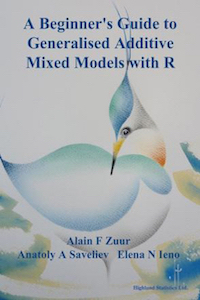www.highstat.com
Highland Statistics Ltd

Latest news

Coming in September 2022:

• Time Series Analysis using Regression Techniques.
• Introduction to GAM and GAMM (frequentist).

See highstat.com for details.# A Beginner's Guide to Generalized Additive Mixed Models with R (2014). Zuur AF, Saveliev AA, Ieno EN

This book begins with an introduction to generalised additive models (GAM) using stable isotope ratios from squid.

In Chapter 2 we explain additive mixed effects using polar bear movement data. In Chapter 3 we apply additive mixed effects models on coral reef data. Ruddy turnstone data are used in Chapter 4 to explain Poisson generalised additive mixed effects models (GAMMs) using the gamm4 package. A simulation study is applied to investigate the effect unbalanced random effects. In Chapter 5 parasite data sampled on anchovy fishes are used to explain overdispersed Poisson GAMM, negative binomial GAMM, and NB-P GAMM models. We briefly discuss generalised Poisson models for underdispersed data.

In Chapters 6 and 7 two-dimensional smoothers are applied on zero-inflated guillemots and harbour porpoise datasets. A short revision of zero-inflated models is included. Gamma GAMMs are applied on two-way nested tree data in Chapter 8. In Chapter 9 binary nested data are analysed using binomial GAMM.

In Chapter 10 we analyse maximum length of cod fishes. The generalised extreme value distribution is used. The data are from a large number of spatial locations and we use INLA to implement spatial correlation. In Chapter 11 sea ducks are analysed using zero-inflated Poisson GAMMs (and GLMMs) with spatial correlation. We again use INLA. Throughout the book we contrast frequentist and Bayesian approaches. All R code is either included and explained in the book or is available from the website for the book.

The title of this book contains the phrase ‘Beginner’s Guide to …’. This does not mean that this book is for the statistical novice and can be read as a stand-alone book. On the contrary, we assume that the reader is familiar with R, data exploration, multiple linear regression, generalised linear modelling, generalised additive modelling, linear mixed effects modelling, and Markov chain Monte Carlo (MCMC) techniques. This is quite a substantial number of statistical techniques. This book is written as a sequel to our Beginner’s Guide to GAM with R and Beginner’s Guide to GLM and GLMM with R books. If you are familiar with the material described in those two books, then the current volume is indeed a ‘Beginner’s Guide’. But if you are not familiar with these techniques then the learning curve may be steep. However, wherever possible we have included short revisions. And we also provide the reader with access to Chapter 1 of Zuur et al. (2012a), which contains an introduction to Markov chain Monte Carlo techniques (see below for access details).

Visit the website of this book to download data, R code, the table of content, and information how to purchase this book.

• Default
• Title
• Date
• Random